# GSEB Solutions Class 7 Maths Chapter 1 Integers InText Questions

Gujarat Board GSEB Textbook Solutions Class 7 Maths Chapter 1 Integers InText Questions and Answers.

## Gujarat Board Textbook Solutions Class 7 Maths Chapter 1 Integers InText QuestionsTry These (Page 2)

Question 1.
A number line representing integers is given below.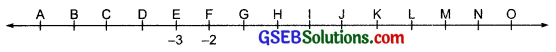– 3 and – 2 are marked by E and F respectively. Which integers are marked by B, D, H, J, M and O?
Solution:
Let us complete the given number line such that integers marked by various alphabets are shown. Therefore,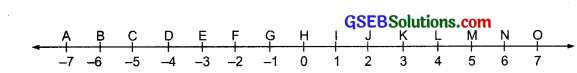The integer marked by B = – 6
The integer marked by H = 0
The integer marked by M = 5
The integer marked by D = – 4
The integer marked by J = 2
The integer marked by O = 7

Question 2.
Arrange 7, – 5, 4, 0 and -4 in ascending order and then mark them on a number line to check your answer.
Solution:
Since
(i) every positive integer is greater than 0.
(ii) every negative integer is less than 0.
∴ – 5 < (- 4) < 0 < 4 < 7
⇒ The required ascending order is: – 5, – 4, 0, 4, 7
Since the integer occurring to the right on a number line is greater than that on the left. And the integer on the left is smaller than that on the right. Now, representing 7, – 5, 4, 0 and – 4 on a number line we get: – 5 < – 4 < 0 < 4 < 7.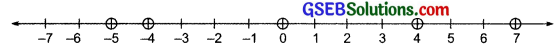Remember : On a number line, when we –

• add a positive integer, we move to the right.
• add a negative integer, we move to the left.
• subtract a positive integer, we move to the left.
• subtract a negative integer, we move to the right.Question 3.
State whether the following statements are correct or incorrect. Correct those which are wrong:
(i) When two positive integers are added we get a positive integer.
(ii) When two negative integers are added we get a positive integer.
(iii) When a positive integer and a negative integer are added, we always get a negative integer.
(iv) Additive inverse of an integer 8 is (-8) and additive inverse of (-8) is 8.
(v) For subtraction, we add the additive inverse of the integer that is being subtracted, to the other integer.
(vi) (-10) + 3 = 10 – 3
(vii) 8 + (- 7) – (-4) = 8 + 7 – 4
Solution:
(i) Correct statement:
Examples:
(a) 55 + 52 = 107
(b) 45 + 71 = 116
(c) 110 + 71 = 181
(d) 145 + 171 = 316
(e) 210 + 103 = 313 107, 116, 181, 316 and 313 are positive integers.

(ii) Incorrect statement – The correct statement is When two negative integers are added, we get a negative integer.
Examples:
(a) (- 72) + (- 57) = – 129
(b) (- 114) + (- 25) = – 139
(c) (- 110) + (- 81) = – 191
(d) (- 39) + (- 31) = – 70
(e) (- 201) + (- 211) = – 412
– 129, – 139, – 191, – 70 and – 412 are negative integers.

(iii) Incorrect statement – The correct statement is When a positive integer and a negative integer are added, we take the difference of the numerical values of the given integers and give the sign of the integer with greater numerical value.
Examples:
(a) (- 52) + 75 = 23
(b) 121 + (- 89) = 32
(c) (- 225) + 105 = – 120
(d) 712 + (- 908) = – 196
(e) 369 + (- 693) = – 324

(iv) Correct statement:
Examples:
(a) The additive inverse of – 38 is 38.
(b) The additive inverse of 29 is – 29.
(c) The additive inverse of – 63 is 63.
(d) The additive inverse of 99 is – 99.
(e) The additive inverse of – 45 is 45.

(v) Correct statement.
Examples:
(a) 58 – 76 means 58 + (- 76) = – 18
(b) 58 – (-176) means 58 + (+ 176) = 234
(c) (- 81) – (45) means (- 81) + (- 45) = 126
(d) – 81 – (- 45) means (- 81) + (+ 45) = – 36
(e) (- 110) – (- 152) means (- 110) + (+ 152) = 42

(vi) Incorrect statement:
Since, R.H.S.= 10 – 3 = 7 and
L.H.S.= (- 10) + 3 = – 10 + 3 = – 7
L.H.S. ≠ R.H.S.
∴ The correct statement is: (-10) + 3 = – 10 + 3

(vii) Incorrect statement.
Since, L.H.S. = 8 + (- 7) – (- 4) = 8 – 7 + 4
= 12 – 7 = 5
and R.H.S. = 8 + 7 – 4 = 15 – 4 = 11
i.e., L.H.S. ≠ R.H.S.
∴ The correct statement is: 8 + (- 7) – (- 4) = 8 – 7 + 4Try These (Page 3)

Question 1.
We have done various patterns with numbers in our previous class. Can you find a pattern for each of the following? If yes, complete them:
(a) 7, 3, -1, -5, _____, _____, _____.
(b) -2, -4, -6, -8, _____, _____, _____.
(c) 15, 10, 5, 0, _____, _____, _____.
(d) -11, -8, -5, -2, _____, _____, _____.
Make some more such patterns and ask your friends to complete them.
Solution: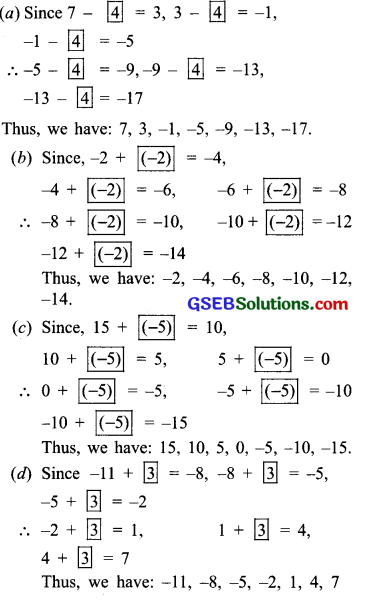Try These (Page 8)

Question 1.
Write a pair of integers whose sum gives
(a) a negative integer.
(b) zero.
(c) an integer smaller than both the integers.
(d) an integer smaller than only one of the integers.
(e) an integer greater than both the integers.
Solution:
(a) (- 15) and 9
Sum: (- 15) + 9 = – 6
[- 6 is negative integer]

(b) – 18 and 18
Sum: (- 18) + 18 = O

(c) (- 6) and (- 4)
Sum: (- 6) + (- 4) = – lo
[- 10 is smaller than – 6 and -4]

(d) 4 and – 6
Sum: 4 + (- 6) = – 2
[(- 2) is smaller than 4 only]

(e) 19 and 21
Sum: 19 + 21 = 40
[40 is greater than 19 and 21]Question 2.
Write a pair of integers whose difference gives
(a) a negative integer.
(b) zero.
(e) an integer smaller than both the integers.
(d) an integer greater than only one of the integers.
(e) an integer greater than both the integers.
Solution:
(a) 3 and – 8
Difference: – 8 – 3 = 11
[- 11 is an integer]

(b) (- 3) and (- 3)
Difference: (- 3) – (- 3) = (- 3) + 3 = O

(c) 5 and 9
Difference = 9 – 5 = 4
[4 is smaller than 9 as well as 5]

(d) 16 and 5
Difference: 16 – 5 = 11
[11 is greater than 5]

(e) 15 and – 6
Difference: 15 – (- 6) = 15 + 6 = 21
[21 is greater than 15 as well as (- 6)]

Try These (Page 10)

Question 1.
Using number line, find:
(i) 4 x (- 8)
(ii) 8 x (- 2)
(iii) 3 x (- 7)
(iv) 10 x (- 1)
Solution:
(i) 4 x (- 8)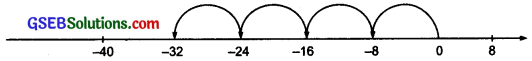From the number line, we have:
(- 8) + (- 8) + (- 8) + (- 8) = – 32
∴ 4 x (- 8) = – 32

(ii) 8 x (- 2)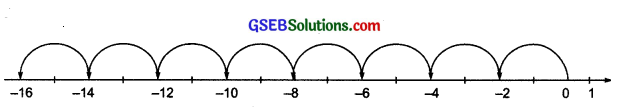From the number line, we have:
(- 2) + (- 2) + (- 2) + (- 2) + (- 2) + (- 2) + (- 2) + (- 2) = – 16
∴ 8 x (- 2) = – 16

(iii) 3 x (- 7)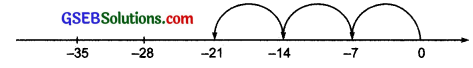From the number line, we have:
(- 7) + (- 7) + (- 7) = – 21
3 x (- 7) = – 21

(iv) 10 x (- 1)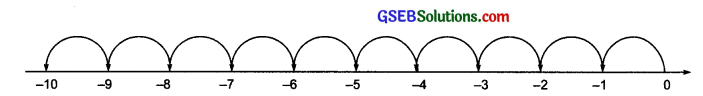From the number line, we have:
(- 1) + (- 1) + (- 1) + (- 1) + (- 1) + (- 1) + (- 1) + (- 1) + (- 1) + (- 1) = – 10
∴ 10 x (- 1) = – 10Try These (Page 10)

Question 1.
Find:
(i) 6 x (- 19)
(ii) 12 x (- 32)
(iii) 7 x (- 22)
Solution:
(i) 6 x (- 19) = – [6 x 19] = –  = – 114
(ii) 12 x (- 32) = – [12 x 32] = –  = – 384
(iii) 7 x (- 22) = – [7 x 22] = –  = – 154

Try These (Page 11)

Question 1.
Find:
(a) 15 x (- 16)
(b) 21 x (- 32)
(c) (- 42) x 12
(d) – 55 x 15
Solution:
While multiplying a positive integer and a negative integer, we multiply them as whole numbers and put a minus sign (- ) before the product, i.e. the product of a positive integer and a negative integer is a negative integer. Therefore,
(a) 15 x (- 16) = – [15 x 16] = –  = – 240
(b) 21 x (- 32) = – [21 x 32] = –  = – 672
(c) (- 42) x 12 = – [42 x 12] = –  = – 504
(d) (- 55) x 15 = – [55 x 15] = –  = – 825

Question 2.
Check if
(a) 25 x (- 21) = (- 25) x 21.
(b) (- 23) x 20 = 23 x (- 20).
Write five more such examples.
Solution:
(a) L.H.S. = 25 x (- 21) = – [25 x 21] = –  = – 525
R.H.S. = (- 25) x 21 = – [25 x 21] = –  = – 525
∵ L.H.S. = R.H.S.
∴ 25 x (- 21) = (- 25) x 21

(b) L.H.S. = (- 23) x 20 = – [23 x 20]
= –  = – 460
R.H.S. = 23 x (- 20) = – [23 x 20]
= –  = – 460
∵ L.H.S. = R.H.S.
∴ (- 23) x 20= 23 x (- 20)
Other examples:
(i) (- 12) x 19 = 12 x (- 19)
(ii) 15 x (- 17) = (- 15) x 17
(iii) 51 x (- 40) = (- 51) x 40
(iv) (- 20) x 25 = 20 x (- 25)
(v) 16 x (- 15) = (- 16) x 15Try These (Page 12)

Question 1.
(i) Starting from (- 5) x 4, find (- 5) x (- 6).
(ii) Starting from (- 6) x 3, find (- 6) x (- 7).
Solution:
(i) Look at the following pattern:
(- 5) x 4 = – [5 x 4] = – 20
(- 5) x 3 = – [5 x 3] = – 15 = – 20 + 5
(- 5) x 2 = – [5 x 2] = – 10 = – 15 + 5
(- 5) x 1 = – [5 x 1] = – 5 = – 10 + 5
(- 5) x 0 = – [5 x 0] = 0 = – 5 + 5
From this pattern, we have:
(- 5) x (- 1) = 0 + 5 = 5
(- 5) x (- 2) = 5 + 5 = 10
(- 5) x (- 3) = 10 + 5 = 15
(- 5) x (- 4) = 15 + 5 = 20
(- 5) x (- 5) = 20 + 5 = 25
(- 5) x (- 6) – 25 + 5 = 30
Thus, (- 5) x (- 6) = 30

(ii) Look at the following pattern:
– 6 x 3 = – [6 x 3] = – 18
– 6 x 2 = – 12 = – 18 + 6
– 6 x 1 = – 6 = – 12 + 6
– 6 x 0 = 0 = – 6 + 6
From this pattern, we have:
– 6 x (- 1) = 0 + 6 = 6
– 6 x (- 2) = 6 + 6 = 12
– 6 x (- 3) = 12 + 6 = 18
– 6 x (- 4) = 18 + 6 = 24
– 6 x (- 5) = 24 + 6 = 30
– 6 x (- 6) = 30 + 6 = 36
– 6 x (- 7) = 36 + 6 = 42
Thus, (- 6) x (- 7) = 42

Try These (Page 12)

Question 1.
Find: (- 31) x (- 100), (- 25) x (- 72), (- 83) x (- 28)
Solution:
We multiply the two negative integers as whole numbers and put the positive sign (+) before the product.
(- 31) x (- 100) = +[31 x 100] = + = 3100
(- 25) x (- 72) = +[25 x 72] = + = 1800
(- 83) x (- 28) = +[83 x 28] = + = 2324

Think, Discuss and Write (Page 14)

Question 1.
(i) The product (- 9) x (- 5) x (- 6) x (- 3) is positive whereas the product (- 9) x (- 5) x 6 x (- 3) is negative. Why?
(ii) What will be the sign of the product if we multiply together:
(a) 8 negative integers and 3 positive integers?
(b) 5 negative integers and 4 positive integers?
(c) (- 1), twelve times?
(d) (- 1), 2 m times, m is a natural number?
Solution:
We know that, if the number of negative integers in a product is even, then the product is a positive integer; if the number of negative integers in a product is odd, then the product is a negative integer.

(i) ∴ The product (- 9) x (- 5) x (- 6) x (- 3) is positive because an even number of negative integers are multiplied.
The product (- 9) x (- 5) x 6 x (- 3) is negative because an odd number of negative integers are multiplied.

(ii)
(a) Positive          [∵ Product of 8 negative integers is positive]
(b) Negative        [∵ Product of 5 negative integers is negative]
(c) Positive           [ ∵ 12 is even and product of even number of negative integers is positive]
(d) Positive           [∵ 2 m is an even number]Try These (Page 18)

Question 1.
(i) Is 10 x [(6 + (- 2)] = 10 x 6 + 10 x (- 2)2
(ii) Is (- 15) x [(- 7) + (- 1)] = (- 15) x (- 7) + (- 15) x (- 1)?
Solution:
(i) Yes, [∵ a x (b + c) = a x b + a x c] 10 x [6 + (- 2)] = 10 x [6 – 2]
= 10 x 4 = 40
And, 10 x 6 + 10 x (- 2) = 60- 20
= 40.
Thus, 10 x [6 + (- 2)] = 10 x 6 + 10 x (- 2)

(ii) Yes, [ ∵ a x (b + c) = a x b + a x c] (- 15) x [(- 7) + (- 1)] = (- 15) x [- 7 – 1]
= (- 15) x (- 8)
= (+) (15 x 8) = 120.
And, (- 15) x (- 7) + (- 15) x (- 1)
= (+) (15 x 7) + (+) (15 x 1)
= 105 + 15 = 120.
Thus, (- 15) x [(- 7) + (- 1)]
= (- 15) x (- 7) + (- 15) x (- 1)

Try These (Page 18)

Question 1.
(i) Is 10 x [6 – (- 2)] = 10 x 6 – 10 x (- 2)?
(ii) Is (- 15) x [(- 7) – (- 1)] = (- 15) x (- 7) – (- 15) x (- 1)?
Solution:
(i) Yes,       [ ∵ a x (b – c) – a x b – a x c]
10 x [6 – (- 2)] = 10 x [6 + 2]
= 10 x 8 = 80.
And, 10 x 6 – 10 x (2) = 60 + (10 x 2)
= 60 + 20 = 80
Thus, 10 x [6 – (- 2)] = 10 x 6 -10 x (- 2)

(ii) Yes,      [∵ a x (b – c) = a x b – a x c]
(- 15) x [(- 7) – (-1)]= (- 15) x [- 7 + 1]
= (- 15) x (- 6)
= (+) (15 x 6) = 90
And, (- 15) x (- 7) – (-15) x (- 1)
= (+) (15 x 7) – (+) (15 x 1)
= 105 – 15 = 90.
Thus, (- 15) x [(- 7) – (-1)]
= (- 15) x (- 7) – (- 15) x (- 1)Try These (Page 19)

Question 1.
By using distributive property, find: (- 49) x 18;
(- 25) x (- 31); 70 x (- 19) + (- 1) x 70
Solution:
(i) (- 49) x 18:
∵ 18 = 10 + 8
= (- 49) x 18
= (- 49) x [10 + 8]
= (- 49) x 10 + (- 49) x 8    [using distributivity]
= – 490 + (- 49)[10 – 2]      [∵ 8 = 10 – 2]
= – 490 + (- 49) x 10 – (- 49) x 2
= – 490 + (- 490) + 98
= – 980 + 98 = – 882

(iii) (- 25) x (- 31):
∵ – 31 = (- 30) + (- 1)
∴ (- 25) x (- 31)
= (- 25) x [(- 30) + (- 1)]
= (- 25) x (- 30) + (- 25) x (- 1) [using distributivity]
= + (25 x 30) + [(25 x 1)]
= 750 + 25 = 775

(iv) 70 x (- 19) + (- 1) x 70:
∵ a x b + a x c = a x [b + c]
∴ 70 x (- 19) + (- 1) x 70
= 70 x [(- 19) + (- 1)] = 70 x [- 20]
= – [70 x 20] = – 1400

Try These (Page 22)

Question 1.
Find:
(a) (- 100) ÷ 5
(b) (- 81) ÷ 9
(c) (- 75) ÷ 5
(d) (- 32) ÷ 2
Solution:
We know that to divide a negative integer by a positive integer, we divide them as whole numbers and then put minus sign (-) before the
quotient.
(a) ∵ 100 ÷ 5 = 20      ∴ (- 100) ÷ 5 = 20
(b) ∵ 81 ÷ 9 = 9         ∴ (- 81) ÷ 9 = – 9
(c) ∵ 75 ÷ 5 = 15        ∴ (- 75) ÷ 5 = – 15
(d) ∵ 32 ÷ 2 = 16        ∴ (- 32) + 2 = – 16

Try These (Page 23)

Question 1.
Find:
(a) 125 ÷ (- 25)
(b) 80 ÷ (- 5)
(c) 64 ÷ (- 16)
Solution:
We know that to divide a positive integer by a negative integer, we first divide them as whole numbers and then put a minus sign (-) before the quotient.
(a) ∵ 125 ÷ 25 = 5       ∴ 125 ÷ (- 25) = – 5
(b) ∵ 80 ÷ 5 = 16         ∴ 80 ÷ (- 5) = – 16
(c) ∵ 64 ÷ 16 = 4         ∴ 64 ÷ (- 16) = – 4Try These (Page 23)

Question 1.
Find:
(a) (- 36) ÷ (- 4)
(b) (- 201) ÷ (- 3)
(c) (- 325) ÷ (- 13)
Solution:
To divide a negative integer by a negative integer, we first divide them as whole numbers and then put a positive sign (+) before the quotient.
(a) ∵ 36 ÷ 4 = 9           ∴ (- 36) ÷ (- 4) = 9
(b) ∵ 201 ÷ 3 = 67       ∴ (- 201) ÷ (- 3) = 67
(c) ∵ 325 ÷ 13 = 25      ∴ (- 325) ÷ (- 13) = 25

Try These (Page 24)

Question 1.
Is (i) 1 ÷ a = 1 and (ii) a ÷ (- 1) = – a for any integer a? Take different values of a and check.
Solution:
(i) Let us take a = – 1, 1, 2, 3, ……..
For a = – 1,
L.H.S. = 1 – (- 1) = – 1 [∵ 1 + 1 = 1]
R.H.S. = 1
i.e. L.H.S. ≠ R.H.S.
For a = 1,
L.H.S. = 1 ÷ a = 1 + 1 = 1
R.H.S. = 1
i.e. L.H.S. = R.H.S.
For a = 2,
L.H.S. = 1 ÷ a = 1 + 2 = $$\frac { 1 }{ 2 }$$ ≠ R.H.S.
For a = 3,
Thus, 1 + a = 1 is true only for a = 1

(ii) Let us take a = 1, 2, 3, …….
For a = 1,
L.H.S. = a ÷ (- 1) = 1 ÷ (- 1) = – 1
R.H.S. = – a = – 1
i.e. L.H.S. = R.H.S.
For a = 2,
L.H.S.= a ÷ (- 1) = 2 ÷ (- 1) = – 2
R.H.S. = a = – 2
i.e. L.H.S. = R.H.S.
For a = 3,
L.H.S. = a ÷ (- 1) = 3 ÷ (- 1) = – 3
R.H.S. = – a = – 3
i.e. L.H.S. = R.H.S.
For a = 7,
L.H.S. = a ÷ (- 1) = 7 ÷ (- 1) = – 7
R.H.S. = – a = – 7
i.e. L.H.S. = R.H.S.
i.e. For every integer, we have a ÷ (- 1) = – a